# Dynamics of particles – problems and solutions

1. Object A with a mass of 6-kg and object B with a mass of 4-kg connected by a cord and pulled by a force of F = 60 N, as shown in the figure below. The coefficient of kinetic friction between the floor and both objects is 0.5 (tan θ = ¾).10 m/s2. What is the magnitude of the tension force?

Known :

Mass of object A (mA) = 6 kg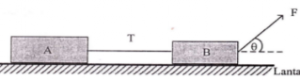Mass of object B (mB) = 4 kg

Force (F) = 60 Newton

The coefficient of kinetic friction between object and floor k) = 0.5

Acceleration due to gravity (g) = 10 m/s2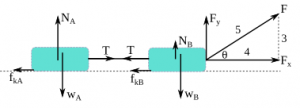Tan θ = 3/4

Wanted : Tension force (T)

Solution :

Horizontal component of force F :

Fx = F cos θ

Fx = (60)(4/5) = (4)(12) = 48 N

Vertical component of force F :

Fy = F sin θ

Fy = (60)(3/5) = (3)(12) = 36 N

The normal force on object A :

NA = wA = mA g = (6)(10) = 60 N

The normal force on object B :

NB + Fy – wB = 0

NB + Fy = wB

NB = wB – Fy = mB g – Fy = (4)(10) – 36 = 40 – 36 = 4 N

The force of kinetic friction between object A and floor :

fkA = μk NA = (0.5)(60) = 30 N

The force of kinetic friction between object B and floor :

fkB = μk NB = (0.5)(4) = 2 N

Calculate the acceleration of both objects :

ΣF = m a

Fx – T + T – fkB – fkA = (mA + mB) a

Fx – fkB – fkA = (mA + mB) a

48 – 2 – 30 = (6 + 4) a

16 = 10 a

a = 16/10

a = 1.6 m/s2

Calculate the tension force :

Object A :

ΣF = m a

TA – fkA = mA a

TA – 30 = (6)(1.6)

TA – 30 = 9.6

TA = 9.6 + 30 = 39.6 N

Object B :

ΣF = m a

Fx – fkB – TB = mB a

48 – 2 – TB = (4)(1.6)

46 – TB = 6.4

46 – 6.4 = TB

TB = 39.6 N

[irp]

2. If the coefficient of kinetic friction between both blocks and floor is 0.2 , what is the acceleration of both objects ? (cos 37o = 0,8, sin 37o = 0,6)

Known :

Mass of object A (mA) = 4 kg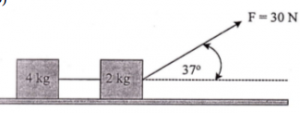Mass of object B (mB) = 2 kg

Force (F) = 30 Newton

The coefficient of kinetic friction between object and floor k) = 0.2

Acceleration due to gravity (g) = 10 m/s2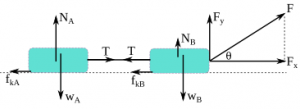cos 37o = 0.8

sin 37o = 0.6

Wanted : Acceleration of both objects

Solution :

The horizontal component of force F :

Fx = F cos θ

Fx = (30)(0.8) = 24 N

The vertical component of force F :

Fy = F sin θ

Fy = (30)(0.6) = 18 N

The normal force on object A :

NA = wA = mA g = (4)(10) = 40 N

The normal force on object B :

NB + Fy – wB = 0

NB + Fy = wB

NB = wB – Fy = mB g – Fy = (2)(10) – 18 = 20 – 18 = 2 N

The force of kinetic friction between object A and floor :

fkA = μk NA = (0.2)(40) = 8 N

The force of kinetic friction between object B and floor :

fkB = μk NB = (0.2)(2) = 0.4 N

Acceleration of both objects :

ΣF = m a

Fx – fkB – fkA = (mA + mB) a

24 – 0.4 – 8 = (4 + 2) a

15.6 = 6 a

a = 15.6 / 6

a = 2.6 m/s2

[irp]

3. Two objects connected by a cord over a pulley as shown in figure below. Mass of object A = mA, mass f object B = mB and the acceleration of block B is a. Acceleration due to gravity is g. What is the tension force on block B.

Solution :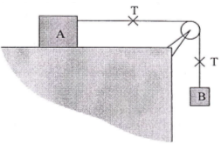The horizontal surface is smooth so there is no friction. The only force that accelerates system is the weight of block B.

System’s acceleration :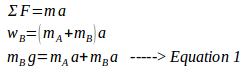The tension force (T) :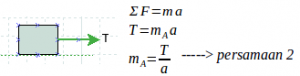Substitute mA in equation 1 with mA in equation 2.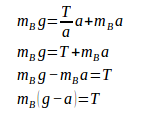[irp]